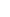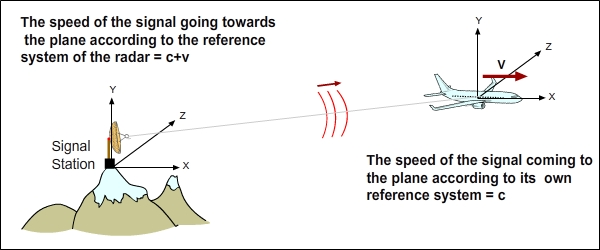7. THINKING WITH (C+V) (C-V) MATHEMATICS IN MINDIt is extremely simple to think with (c+v) (c-v) mathematics in mind. The figure below depicts a situation where a station on top of a mountain communicates with a plane. There are two frames that are in motion relative to each other. We are going to focus on this part to find the signal speeds. Who sends the signal? Who receives it?In the figure, the signal moves towards the plane. Relative to the reference system of the station that sends the signal, the speed of the signal it sends is, relative to itself, c+v (as it is the side sending the signal). If the plane went towards the station, the signal speed would be c-v. The speed of the same signal relative to the reference system of the plane is c (as the signal comes towards it).In this second figure, however, the plane is the one sending the signal. Who sends the signal? The plane. Then the speed of the signal that plane sends to the station on the ground relative to the planes reference system is c+v. Who receives the signal? The station on the ground. Then the speed of the signal coming to the station relative to the stations reference system is c.We should always remember the principles. In case we come across a difficulty while examining an event, it is possible for us to deal with that difficulty by assuming that any of the reference systems stands still and that the other is in motion.In the above figure, there are three planes moving at different speeds and in different directions relative to the coordinate system in the figure. Relative to the reference system of the Plane 1, what is the speed of the signal that Plane 2 sends to Plane 3?Answer: The signal, independent of the speed of the plane that sends it, will go to its target, Plane 3, at c speed relative to the reference system of Plane 3. The speeds of Plane 1 and Plane 3 relative to each other is v1 + v3. Therefore, the speed of the signal relative to the reference system of Plane 1: c' = c + v1 + v3. We can see that we can find the answers fairly quickly when we apply the rules and the principles.Now we have come to quite an interesting point. Lets think that our source of the signal is a bulb and that there are many objects going in different directions at different speeds around it. The lights that the bulb emits relative to its reference system will go to each moving object at a different speed. This reality is the inevitable result that (c+v) (c-v) mathematics imposes upon us at first hand. While all these are taking place, we need to pay attention to how the speed of light constant c is meticulously preserved. Relative to each reference system, the speed of the light coming to each is c. Id like to you to notice this marvelous and astonishing event we have come across.Below are two sportsmen running and there is light coming to them from the bulb. The speed of the runner at the back is v1 and he runner in the front is v2. In this case, how will (c+v) (c-v) mathematics will occur?By thinking that the speeds of the runners are v1 and v2, relative to the reference system of the bulb, it is, of course, correct to say, as a correct approach, that the light will travel to the runner at the back at c+v1 speed and to the one in the front at c+v2 speed, but it would only be an overall approach. If we examine it individually, it is not the case at all. Relative to the reference system of the bulb, the speeds of the parts of their bodies are different while they are running. Their trunks, heads, hands, feet, arms, legs, and even strands of their hair streaming while they are running are different targets for the lights emitted from the bulb. Therefore, lights emitted from the bulb will travel to each part of their body at a different speed.Here, we see two important logical conclusions.Each part constituting an object is a different object. Each element that constitutes the object is a different object and a different reference system according to the rules of (c+v) (c-v) mathematics. I will touch upon this issue again soon, so I will just put it aside at this stage.The other conclusion is the following: (c+v) (c-v) mathematics says that we should not see light as a whole. The thing we call light is a cluster of almost an infinite number of electromagnetic waves (photons). (c+v) (c-v) mathematics tells us to consider not the cluster but the elements forming the cluster; that is, to think with photons in mind, not light. A single photon and a single point of arrival as a target. Lets deepen our understanding of the topic a bit more by asking a few questions:Question: What emits the photons coming out of the bulb? Answer: When the atoms constituting the filament of the bulb gets warmer, photons begin to spread.Question: What is the target of the photon emitted? Answer: An atom on another object.Therefore, (c+v) (c-v) mathematics actually describe the rules related to the speed of the photon in the electromagnetic interaction between two atoms. The reference system that sends the light (photon) is the atom emitting the photon and the target of the photon emitted, namely the target reference system, is the atom to receive the photon. If two atoms are in motion relative to each other, the photon emitted behaves in the way described with (c+v) (c-v) mathematics.It is, of course, possible to go deeper in thoughts. You may also think that the electromagnetic interaction is, as a matter of fact, carried out by electrons and therefore that (c+v) (c-v) mathematics should be related to the electromagnetic interaction between two electrons. I preferred to go down to the level of atoms. Normally, in order for us to think with (c+v) (c-v) mathematics in mind, we do not need to go into atomic scale or the internal structure of atoms. As in the example of the runners, thinking with objects and electromagnetic waves that make up light instead of light should be more than enough.Naturally, a question emerges on its own at this stage: Is it possible for a photon to know which object is its target, to use that objects reference system as base, to know if its target is in motion or not, and to travel at a speed of c relative to its targets reference system? Id like to answer this question with another question: Are the principles that we used as a base at the beginning of the book wrong? We have reached (c+v) (c-v) mathematics with these principles. If we think these principles are correct, these questions that we have just asked and whose answers seem impossible to get must also have answers and they do have answers indeed. I included my own opinions regarding this topic in the third and fourth chapter of the book. For now, there are more essential topics and Id like to broach those topics.